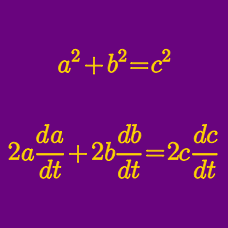Calculus

# Related Rates - Word Problems

A $13\text{ feet}$ long ladder is leaning against a wall and sliding toward the floor. If the foot of the ladder is sliding away from the base of the wall at a rate of $17\text{ feet/sec},$ how fast is the top of the ladder sliding down the wall (in feet/sec) when the top of the ladder is $5\text{ feet}$ from the ground?

At time $t=0 \mbox{ s}$, the radius of a circle is equal to $19 \mbox{ cm}$. The radius of the circle increases at a rate of $\frac{1}{2} \ \mbox{cm/s}$. The rate of change of area at $t=18 \mbox{ s}$ is equal to $m\pi \ \mbox{cm}^2\mbox{/s}$, where $m$ is a positive integer. What is the value of $m$?Throwing a stone in a calm lake forms concentric ripples, as shown in the above picture. If the radius of one ripple increases at the rate of $10 \text{cm}$ per second, what is the rate of increase of the area of the ripple $2$ seconds after a stone is thrown (in $\text{cm}^2/\text{s}$)?

During a rainstorm, the area of a circular pool of water increases at the rate of $4000\pi\text{ in}^2\text{/sec}.$ At what rate is the radius expanding (in inches/sec) when the radius is $200\text{ inches}?$

A point is moving along the parabola $x+5y^2=0$ toward the origin with $x$-coordinate increasing at the rate of $5\text{ units/s}$ and $y$-coordinate positive. How fast is the point moving in terms of the remaining distance to the origin as it passes through the point $(-5,1)$ on the parabola?

×